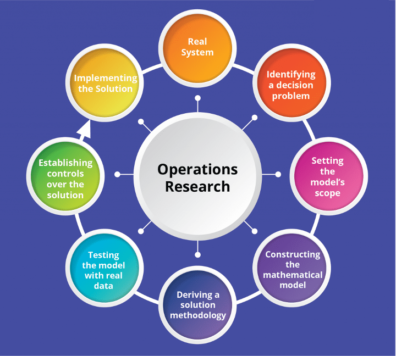Voiland College of Engineering and Architecture Engineering and Technology Management

# E M 540 Operations Research and Analytics for Managers

#### Course Description

Operations Research (OR) extends math modeling to managerial decisions and operational problems which have many solutions. OR uses these models to better understand the options available to the manager using data and analytics. OR and analytical techniques include a collection of optimization models to help rationalize and guide the manager towards the ‘best solution’ among the many possible satisfying solutions. This course introduces the student to a number of models, which have been proven effective in solving certain classes of managerial problems in engineering management systems and operations. The student will see the rationale behind the technique and understand how to apply the tools. Techniques covered in the course include linear and nonlinear programming, network optimization, goal programming, decision trees, and simulation.

#### Course Objectives

• Students will be able to recognize problems in real-world situations where operations research models can be applied.
• Students will understand models and techniques, formulation and results of an analytical or mathematical nature that aid decision-making.
• Students will be able to apply models and techniques in their industry or area of work.
• Students will gain a perspective that integrates management, computer science and mathematics to solve the new challenges of modern complex systems.

#### Learning Outcomes

• L1. Distinguish types of models from optimization, decision analysis, simulation, and others,and recognize their limitations and applicability
• L2. Identify, formulate, and solve complex engineering and technology management problems by applying mathematical and computational modeling of real-world problems, including fundamental applications of those techniques in industry.
• L3. Using case studies, analyze problems and communicate solutions in a group learning context.
• L4. Use a statistical software package for computations with dataFaculty Contact: Luna Magpili
(luna.magpili@wsu.edu)
Semester Credits: 3
Prerequisites: NoneThis course can be used to fulfill the following requirements:

#### Course Topics

• Linear Programming
• Sensitivity Analysis
• Integer, Mixed Integer Programming
• Network Models
• Goal Programming
• Nonlinear Programming
• Probability Concepts
• Descriptive vs Prescriptive Analytics
• Simulation Modeling
• Decision Analysis and Decision Trees
• Integrating Models
• Using Simulation with Decision Trees